telescopeѲptics.net          ▪▪▪▪                                             CONTENTS

13.3. Eye aberrations   ▐    13.4.2.  2nd order defocus and astigmatism, off-axis

# 13.4. MONOCHROMATIC EYE ABERRATIONS

PAGE HIGHLIGHTS
Axial defocus   • Axial astigmatism

The list of most significant monochromatic eye aberrations includes defocus, astigmatism, coma, spherical aberration, and trefoil, as well as optical roughness, caused by local surface deformations and lack of media homogeneity. Even a good average eye generates enough of a wavefront error to require pupil diameter smaller than 2mm for near diffraction-limited level (~λ/14 RMS wavefront error for 0.55μ wavelength).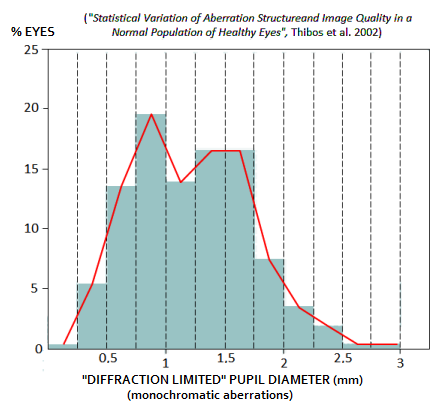Graph at left shows statistical distribution of the "diffraction limited" pupil diameter according to a study. It indicates that most people fall between 0.5mm and 1.7mm, with the rough average at about 1.2mm. The combined error increases and decreases progressively with the pupil diameter.

Due to the typically highly asymmetrical, irregular wavefront shapes produced by human eye, especially at larger pupil sizes, the method of choice for assessing eye aberrations are Zernike circle polynomials. However, Zernike scheme commonly used in ophthalmology - the ANSI standard Zernike expansion - is different from those used in assessing telescope aberrations. It is graphically presented as a pyramid resulting from Zernike term expansion as a function of radial order n and angular frequency m, with the latter being numerically positive for cosine function of θ, and negative for the sine function (FIG. 225; the relation between the sign of m and the function is illustrated on FIG. 30, 4). The terms are ordered and enumerated according to the specifically defined ordering number j.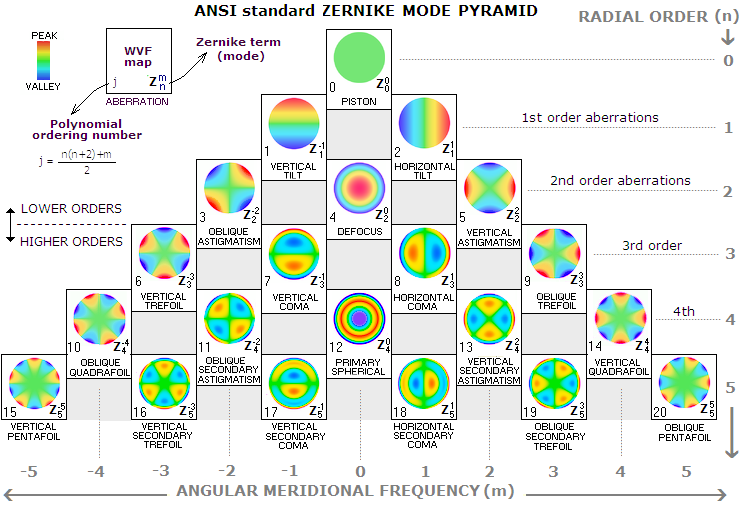FIGURE 225
: Zernike terms expansion pyramid is a function of term's radial degree (or order) n and azimuthal frequency m. It is the basis for classifying aberrations as lower (n≤2) and higher-order (n>2) in ophthalmology. Shown are the top 20 terms. At left: associated Zernike terms and names of aberrations; the so called j-number (commonly referred to as mode), the polynomial ordering number, is dependant on n and m, determining position of the term in the Zernike terms' expansion (note that ordering number j is defined differently here than in Noll's indexing scheme, and also differs from Wyant's indexing scheme). In full notation, each term is identified with Z(ρ,θ), but the coordinates are commonly omitted, and the terms are identified in double-index notation by Z. Data presentation, however, does not use the Zernike term itself, but its portion determining the actual level of aberration - Zernike expansion coefficient (also: Zernike aberration coefficient). The coefficient, whose absolute value equals the RMS wavefront error,  is denoted by z;  alternately, it can be written as zn,m ; as z(n,m), or only (n,m). Finally, in so called single-indexing notation, a term can be identified by its polynomial (j) number, as Zj, and so can the corresponding coefficient, as zj (Zernike coefficient, denoted by z here, is most often denoted by c or a in ophthalmologic literature)

Piston and tilt (distortion) terms usually are omitted from consideration, the former due to being zero for single-pupil imaging, and the latter being an aberration affecting point image location, not its quality. The common division of the expansion terms is on the lower, or 2nd order aberrations (defocus and astigmatism), and higher-order aberrations. The latter include unlimited number of terms, but usually limit to orders 3 to 5, or 3 to 6, since yet higher orders are generally negligible.

Following the above division of the Zernike expansion adopted in ophthalmology, monochromatic eye aberrations are addressed as: (1) lower-order aberrations, with the Zernike radial order n<3, and (2) higher-order aberrations, with n≥3. This division is particularly appropriate for the concept of eye aberrations, because the lower-order aberrations - and particularly the 2nd order aberrations, defocus and primary astigmatism - are the most significant contributors to the overall magnitude of eye aberrations (remaining lower-order forms, piston and tilt, or distortion, are usually ignored, with the former being not an aberration with a single imaging pupil, and the latter being not a point-image quality aberration).

Monochromatic aberrations in an optical system are commonly separated into axial, occurring in the field center, and off-axis, occurring in the outer field as a result of the obliqueness of incident light pencils. Correction of the former is the primary goal, since objects are generally observed in the central field. Unlike typical telescope systems, which - if properly made and aligned - consist of rotationally symmetrical surfaces centered around optical axis, the eye operates in inherently misaligned mode, with surfaces lacking rotational symmetry to a significant degree. As a result, typical off-axis aberrations in a telescope (e.g. coma and astigmatism), are commonly present in the fovea, as well as in the outer retina (the difference being that the latter originate mainly from the obliquity of incident light pencils, reaching significantly larger magnitude at large incident angles) . Thus terms axial and off-axis, when associated with eye aberrations, do not imply specific group of aberrations, as with telescope systems, rather any aberrations appearing in the central retina, as a result of eye imperfections.

Axial (also central, or foveal) eye aberrations are the primary limitation to its proper functioning both, in daily life and as a part of optical train involving use of a telescope. Off-axis (also oblique, or abaxial) eye aberrations are comparatively unimportant, but will be also addressed, since they can affect quality of extended object images, or extended fields projected onto the retina.

Generally, the magnitude of aberration contribution decreases with increasing Zernike radial order. Second order aberrations account for roughly 90% of total aberration of the average eye. The most significant higher-order terms are 3rd order coma and trefoil, and 4th order spherical aberration, as summed up in the table below (as mentioned before, zero-order piston is not an aberration for image formed by a single aperture, and 1st order tilt/distortion is not a point-image quality aberration; for that reason, they are usually omitted from evaluating  eye aberrations).

 RADIAL ORDER (n) ABERRATION ZERNIKE TERM ZERNIKE COEFFICIENT ZERNIKE CIRCLE POLYNOMIAL Double-index notation (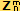) Single-index notation (Zj) Double-index notation Single-index notation (zj) 2ND Oblique primary astigmatism Z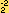Z3 z2,-2 z3 ρ2sin2θ Defocus ZZ4 z2,0 z4 2ρ2-1 Vertical primary astigmatism ZZ5 z2,2 z5 ρ2cos2θ 3RD Vertical trefoil ZZ6 z3,-3 z6 ρ3sin3θ Vertical primary coma ZZ7 z3,-1 z7 (3ρ3-2ρ)sinθ Horizontal primary coma ZZ8 z3,1 z8 (3ρ3-2ρ)cosθ Oblique trefoil ZZ9 z3,3 z9 ρ3cos3θ 4TH (the most significant 4th order aberration) Primary spherical aberration ZZ12 z4,0 z12 6ρ4-6ρ2+1

Following the main division of monochromatic eye aberrations on lower and higher orders, according to the ANSI Zernike terms expansion, the text continues with more detailed description of 2nd order eye aberrations, on axis (foveal) as well as off-axis. Next page addresses higher-order eye aberrations.

13.4.1. 2ND ORDER ABERRATIONS: AXIAL DEFOCUS AND ASTIGMATISM

As already mentioned, defocus and 2nd order (primary) astigmatism account for roughly 90% of the total monochromatic aberration of the average eye. Among the two, defocus is clearly dominant, being up to several times larger in magnitude.

Axial (foveal) defocus and astigmatism are addressed first, followed with a description of off-axis extent of these two aberration forms.

Axial defocus

In ophthalmology, defocus is second-order aberration, denoted with the Zernike term Zand Zernike (expansion) coefficient z2,0 (short notation may only use its n and m integers, i.e. 2,0). Its j-number, or mode, is 4, which means that the term can also be identified as Z4, and the coefficient as z4. As mentioned, defocus is by far the largest single inherent eye aberration. Luckily, it is also the easiest to correct - a simple positive or negative lens placed in front of eye will do.

Axial eye defocus is caused primarily by the mismatch between optical and/or accommodative power of the eye and the size of vitreous body; other contributing factors can be weakened muscles surrounding eye lens, and/or lack of elasticity of the lens itself. The more the separation between cornea and retina deviates from 23-24mm, the more likely significant axial defocus error; in general, larger eye tends to suffer from myopia (short focus for distant objects, improving with reducing object distance), and smaller eye from hyperopia (long focus for distant objects, worsening with the reduction in object distance). Overgrowth of the vitreous body - either as a whole, or as an elongation along visual axis, or as an outward deviation in the concavity of the rear retinal wall - is much more common than undergrowth, hence myopia is also more frequent than hyperopia.

Farther off-center, retinal shape (contour) - which is generally prolate ellipsoid, but of varying vertex radius, conic and local deviations - is also a factor that can be significant in determining the magnitude of defocus error, and so are the Petzval and astigmatic field curvature. Together with optical power of the eye, these are the factors determining the magnitude of off-axis defocus error, which is addressed in the subsection on off-axis eye aberrations.

Following table is a short summary of defocus-related terms and relations (z4 value obtained from the P-V wavefront error is in wavelengths, and to obtain its value in microns needs to be multiplied with the wavelength expressed in microns).

 DEFOCUS Zernike z4 to diopters conversion (for z4 in microns) (for z4 in wavelengths) z4 to P-V wavefront error conversion termcoefficient zn,m order (n) j-number single-index notation (term/coefficient) D=-16z4√3/P2 =M* D=-16λz4√3/P2 P-V=z4√12 Zz2,0 2 4 Z4 / z4 * Common notation for so called "spherical equivalent", ophthalmic prescription for a correcting lens in diopters;  P=pupil diameter

Average accommodation power of the eye decreases with age, from 10-15 diopters at age 20, to 1-2 diopters at age 60 (FIG. 221 bottom left). Accommodation power in diopters can be expressed simply as 1/S, with S being the eye-to-object separation in diopters (i.e. in units of 1 meter); hence accommodation power of 10 diopters implies ability of eye lens to refocus from zero (object at infinity) to 0.1m, and 1 diopter accommodation power implies ability to refocus from infinity to objects 1m away. The smallest distance at which eye can sharply focus is referred to as the least distance of distinct vision or, more simply, near-focus distance.

Diopter (or dioptre, abbreviated by D), expressed as D=1/f, in which f represents the lens power (focal length) in meters, is used as a measure of longitudinal defocus. Since the effective focal length of the eye (the f.l. that the eye would have with air as imaging medium) is fE~17mm, or ~0.017m,  its diopter is ~59. The larger diopter, the stronger optical power, i.e. the shorter focal length. The difference between the optical powers of rays from the central and outer zones of eye pupil indicates the amount of defocus. A 2D defocus caused by a different optical power of the outer zone of the eye lens means that the outer zone diopter is 61. In effect, it is by ~1/30 of the proper focal length shorter, as if formed by rays falling onto the eye lens being slightly converging. Negative diopter indicates that the outer zone focuses longer. Thus -2D defocus indicates 57D power for the outer zone of the eye lens, or focal length by 1/30 of the central rays' focal length longer, as if coming - approximately - from a point not at infinity, but located at 30 eye lens' focal lengths - or 2 diopters - in front of the eye.

Formaly, diopter prescription with eye defocus indicates the power of lens needed to correct the aberration. So if eye defocus is, for instance, 3 diopters, making its focal length 62 diopters, it will be corrected by putting a -3D lens (negative, with focal length of -1/3 meter) in front of the aberrated eye. This is due to the combined focal length being approximated with a thin lens equation, as 1/fC=(1/fE) +(1/fG), which for focal lengths in diopters becomes DC=DE+DE, with subscripts C, E and G are standing for "combined", "eye" and "(eye)glasses", respectively.

Defocus expressed in diopters, D*, can be converted into longitudinal defocus L, as L~D*fE/60, and from it into P-V wavefront error W=L/8F2 which, with the focal ratio F~17/P, where P is the eye pupil diameter, can be written as W=LP2/2300. Substituting D*fE/60 for L, and taking fE~17mm, in terms of defocus error in diopters D* it becomes W=D*P2/8100. So, for instance, one diopter of defocus results in 0.23 wave P-V wavefront error at 1mm pupil, and 5.7 waves at 5mm pupil (for 550nm wavelength). The corresponding RMS wavefront error is smaller by a factor 1/12, and equals absolute value of the Zernike expansion coefficient. Denoting RMS error with ω, it can be written as ω=|D|P2/28,000. Solving for P and ω=0.000041mm (diffraction limited RMS error for 0.00055mm wavelength) determines the limit for diffraction limited defocus error in diopters as D*~1.15/P2. That gives 0.29 diopters defocus limit at 2mm pupil diameter, and 0.13 diopters at 3mm pupil diameter.

It is safe to say that relatively few individuals have natural eyesight with such a low level of defocus error. Looking at a few recent studies, inherent eye defocus errors are quite common, and often quite large. For instance, among 109 "normal" participants aged 21 to 65 years (41y average), defocus, or spherical refractive error was between +6D and -12D (-3D mean, +/-3D standard deviation). The average eye here (Porter et al. 2000)is far from diffraction limited even at 1mm pupil diameter. Another study on 100 students of Indiana University School of Optometry, aged 22-35y (26.1y average), had nearly identical overall defocus error, +5.5D to -10D range, with -3.1D mean +/-3D SD (Indiana Aberration Study, Thibos et al. 2002). These numbers indicate that eye defocus error does not increase significantly with age. In the Indiana Aberration Study, only about 20% of participants had defocus error less than 1D. Majority had myopia (short sight, numerically negative defocus error), which is representative of the population in general.

Yet another group of students, this time from Murcia University, Spain (59 students, 20-30y old, 24y average) had significantly lower defocus error at +1.3D mean +/-1.7D SD (Castejan-Mochan et al. 2002). These numbers, on the other hand, indicate that there may still be substantial random variability in groups of this size.

Between about 3D average defocus error in the first two, and 1.3D in the third study, a broad population average may be at 2-2.5D level. With the corresponding RMS wavefront error ω=DP2/163 in microns, the uncorrected average eye would be diffraction limited defocus-wise (for 0.55μ wavelength, thus ω=0.55/180) roughly at a pupil diameter P of 0.7mm.

However, regardless of the magnitude of defocus error in the eye, it does not affect the telescope observer: s/he corrects for it by defocusing the eyepiece by an offsetting amount.

It is another focusing-related eye function that can have the effect on perceived image quality in a telescope - specifically, quality of the outer field. It is the eye accommodation power.

The image field produced by telescope objective is usually curved, not seldom quite strongly. Depending on the the sign of curvature, it causes outer field image points to be progressively closer, or farther away from the eyepiece. This, in turn, causes these light pencils to exit the eyepiece slightly diverging (when closer) or converging, i.e. appearing as coming from a finite distance. In order to bring these pencils into focus, eye has to accommodate, primarily by changing the shape of eye lens. The eyepiece itself can produce strong image curvature, which can make curvature of the final best image surface either stronger or weaker than that of the objective's image.

Neglecting effect of the eyepiece - whose specific image curvature is commonly unknown - the power of accommodation required due to the curvature of image formed by the objective can approximated using basic paraxial relation (Eq. 1.4). Substituting f+(h2/2R) for object distance, with f being the eyepiece focal length, h being the point height in image space and R the radius of image curvature, gives the apparent point distance p as:

p=2R(f/h)2+f.

With f and h in mm, the corresponding accommodation power in diopters is 1000/p. For, say, image curvature radius R=100mm, eyepiece f.l. f=10mm and point height h=5mm (which is at a field edge of the standard 10mm 50° AFOV eyepiece), p=810mm and needed power of accommodation is 1.2 diopters. This level of accommodation is achievable for most people, and quite easy for younger individuals. However, with significantly wider field, or significantly stronger field curvature, or with the eyepiece significantly adding to the final image curvature, older individuals may not be able to see the outer field clearly without adjusting the focuser.

The specific form of image curvature, i.e. whether it is concave or convex toward the eye, is an additional factor that influences how demanding is accommodation. It is harder for the eye to focus a converging beam - such as one coming from off-axis point farther away than the center (i.e. from outer portion of image surface convex toward the eye - since it is not a natural geometry, requiring eye lens to weaken its surface curvatures beyond those needed for focusing near-collimated beam.

The next largest axial aberration of the average eye is primary astigmatism. It is mainly the combination of eye's defocus and astigmatism that is mainly responsible for often grotesquely deformed, stretched out diffraction patterns produced by the eye at larger pupil sizes. As mentioned, eye defocus error is inconsequential for visual observing with a telescope, which acts as a corrective aid. Unfortunately, this automatic correction does not extend to eye astigmatism.

Axial astigmatism

Like defocus, primary astigmatism in ophthalmology is second-order aberration, denoted with the Zernike term Zand Zernike (expansion) coefficient z2,2 for vertical astigmatism (denoted as J0 or J180 when expressed as prescription cylinder power in diopters), and Zfor oblique astigmatism, with Zernike (expansion) coefficient z2,-2, denoted by J45 as the corresponding corrective prescription power in diopters. The j-number, or mode, for the former is 3, and for the latter 5.

Astigmatism in human eye can be axial, which most often originates at the cornea, prone to relatively weak cylindrical deformations, or regular off-axis astigmatism. The extent of the former is determined by the severity of corneal deformation, while the latter, in general, becomes progressively larger with the increase in off-axis angle (i.e. with the increase in retinal eccentricity).

 2ND ORDER (PRIMARY) ASTIGMATISM Zernike z3 and z5 to diopters conversion (for z4 in microns) combined terms z3 and z5 to P-V wavefront error conversion termscoefficients zn,m order (n) j-number single-index notation (term/coefficient) D=-8z3√6/P2 = -J45 D=-8z5√6/P2 = -J0/180 J=J0+J45 =(J02+J452)1/2 P-V=z3√24 P-V=z5√24 Zand Zz2,-2 and z2,2 2 3 and 5 Z3 / z3 and Z5 / z5

Astigmatism Zernike coefficients z2,-2 and z2,2 converted to diopters (i.e. astigmatism wavefront to longitudinal error) for the purpose of ophthalmic glasses prescription, are commonly denoted as J45 and  J0 or J180, respectively (not to confuse with the j-number). The former is termed oblique astigmatism, and the latter vertical/horizontal astigmatism (in ophthalmic terminology, the latter is called with-the-rule and against-the-rule astigmatism, with the former - prevalent in children - being caused by the vertical wavefront radius stronger than horizontal, and the latter - prevalent in adults - the other way around).

Note that oblique astigmatism related to the Zernike term is not to be confused with the regular off-axis astigmatism on the retina, frequently also referred to as oblique astigmatism. The former is so called asymmetrical (or antisymmetrical) term used to describe astigmatic wavefront with the two main astigmatic meridians at an angle different than 90°.  In other words, when the two astigmatic corneal axes are not perpendicular, the oblique J45 mode is used to better approximate the shape of resulting wavefront deviation. When both, J0 and J45 terms are present, their combined value is given by the square root of the two values squared: J=(J02+J452)0.5. The resulting deviation from 90°-angle between two meridians - called axis - is given by 0.5[tan-1(J45/J0)] or, for that matter, by 0.5[tan-1(z3/z5)].

Axial astigmatism in the average eye is only a fraction of its axial defocus error. Turning to the same three studies cited with defocus, the astigmatism-to-defocus ratio ranges from about 1:10 (Indiana Aberration Study, as the mean longitudinal error in diopters) and 1:7 (Porter et al. as the absolute RMS wavefront error) to 1:3.6 (Castejan-Mochan et al.), the last one as the mean Zernike expansion coefficient. None of the studies are directly comparable, the first having the aberration expressed as longitudinal (in diopters), as opposed to RMS wavefront in the second, and Zernike coefficients in the third study (Zernike coefficients numerically equal the RMS wavefront error but, unlike it, can have negative value as well, hence different - generally smaller - mean value).

Longitudinal astigmatism error in diopters J=0.3D from the Indiana study can be converted back to the corresponding combined mean Zernike expansion coefficient for astigmatism as zAc=JP2/166, (assuming J~J0, with the latter usually amounting to most of J value) where P is, as before, eye pupil diameter. For defocus, conversion relation is z2,0=D*P2/163, hence defocus coefficient vs. that for astigmatism is larger by a factor of 2 for given longitudinal error (this merely reflects the difference in the P-V/RMS error ratio between the two aberrations; since the P-V error expression for given longitudinal error is identical for defocus and primary astigmatism, so is the P-V wavefront error for given defocus in diopters, as specified for defocus error above). Thus, in terms of Zernike coefficients, the astigmatism-to-defocus error ratio in the Indiana study is 1:14, or nearly four times smaller than 1:3.6 (also in terms of Zernike coefficients) in Castejan-Mochan et al.

The ratio in the third study, Porter et al., is not directly comparable these two studies, since mean errors were calculated from the absolute values of Zernike coefficients, i.e. from the RMS values. Since that excludes partial offset between positive and negative coefficient values, this average value reflects the actual average of error magnitude, and will give a higher mean value. It can't be specified by how much without knowing the raw data. Absolute average will be greater for both, defocus and astigmatism, but less so for defocus, which is mainly myopic, with negative coefficient values. Values more comparable to a mean of absolute values are usually those of the standard deviations from mean for both, positive and negative values. Taking standard deviation from the Indiana and Spanish study instead of the means, gives astigmatism-to-defocus ratio for the two of 1:11 and 1:2.3, respectively, which should be at least roughly in the same form as the 1:7 ratio from Porter et al.

These three ratio values are too widely separated to indicate, at least approximately, astigmatism-to-defocus ratio in the general population. The values clearly indicate that some study populations were biased toward atypical averages either for defocus or astigmatism, or both. Averaged astigmatism-to-defocus ratio for broad population is very likely between 1:10 and 1:3, still too broad to be useful. Including ratio values for sample populations from two other studies gives somewhat better idea of the average ratio value: 5.1 (Cheng et al. 2004, 74 subjects aged 21-40 years, -2.50D+/-2.25D mean defocus error and -0.70D+/-0.54D primary astigmatism, the latter subject to 0.5 correction factor for conversion to wavefront errors), and 1:6.6 and 1:2.4 for ~23 and ~60 years of age, respectively (McLellan et al. 2001, 38 subjects aged 23-65 years, as a ratio of mean astigmatism to mean spherical equivalent refractive error), place the simple ratio average for these five studies between 1:4 and 1:5. However, the last study indicate that the ratio value varies significantly depends with age.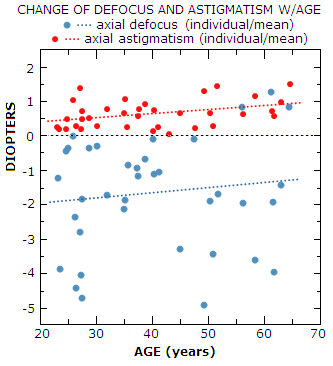In part, these ratio discrepancies are due to the variations in the level of astigmatism of sample populations: average axial astigmatism is over 50% greater in Castejan-Mochan et al. vs. Porter and al., with the latter having it about 1/3 higher than Thibos et al. Thus nearly half of the discrepancy in the astigmatism-to-defocus ratio comes from the difference in the level of astigmatism from one study population to another.

Sample-to-sample variations in the average defocus error are even more significant. For instance, range of defocus error was significantly wider for participants in Porter et al. and Indiana study (+6D to -12D and +5.5D to -10D, respectively), than in Cheng et al. (+1.25 to -8.25D) and McLellan et al. (+0.5D to -6D, with a single extreme positive subject with +4.6D excluded from regression analysis; plot based on McLellan et al. is shown at left, with raw defocus values substituted by "spherical equivalent refractive error", somewhat modified by combining it with spherical aberration present, but gravitating toward the raw values).

Obviously, this degree of random variations in the average axial defocus and astigmatism in study populations will inevitably result in a wide range of their ratio value.

It shows that these sample sizes, despite being relatively large by the standards applicable to eye aberration research, are still to small to be reliable indicator of the average magnitude of these two major axial eye aberrations in the general population. Considering age dependence for the two aberrations implied by McLellan et al., the average population astigmatism-to-defocus ratio, very approximately, could range from 1:7 in the early twenties to about 1:3 in late fifties. As the above graph indicates, most of the increase in the ratio value is due to the increase in averaged astigmatism, which more than doubles from age 20 to age 60. Decrease in averaged defocus with age is comparatively small.

Since defocus and axial astigmatism make about 90% of the total axial aberration of the average eye, astigmatism alone accounts for about 15-30% of it, less in younger population, and more in the older one. Again - and as the above graph shows - individual deviations from the overall average are wide and unpredictable.